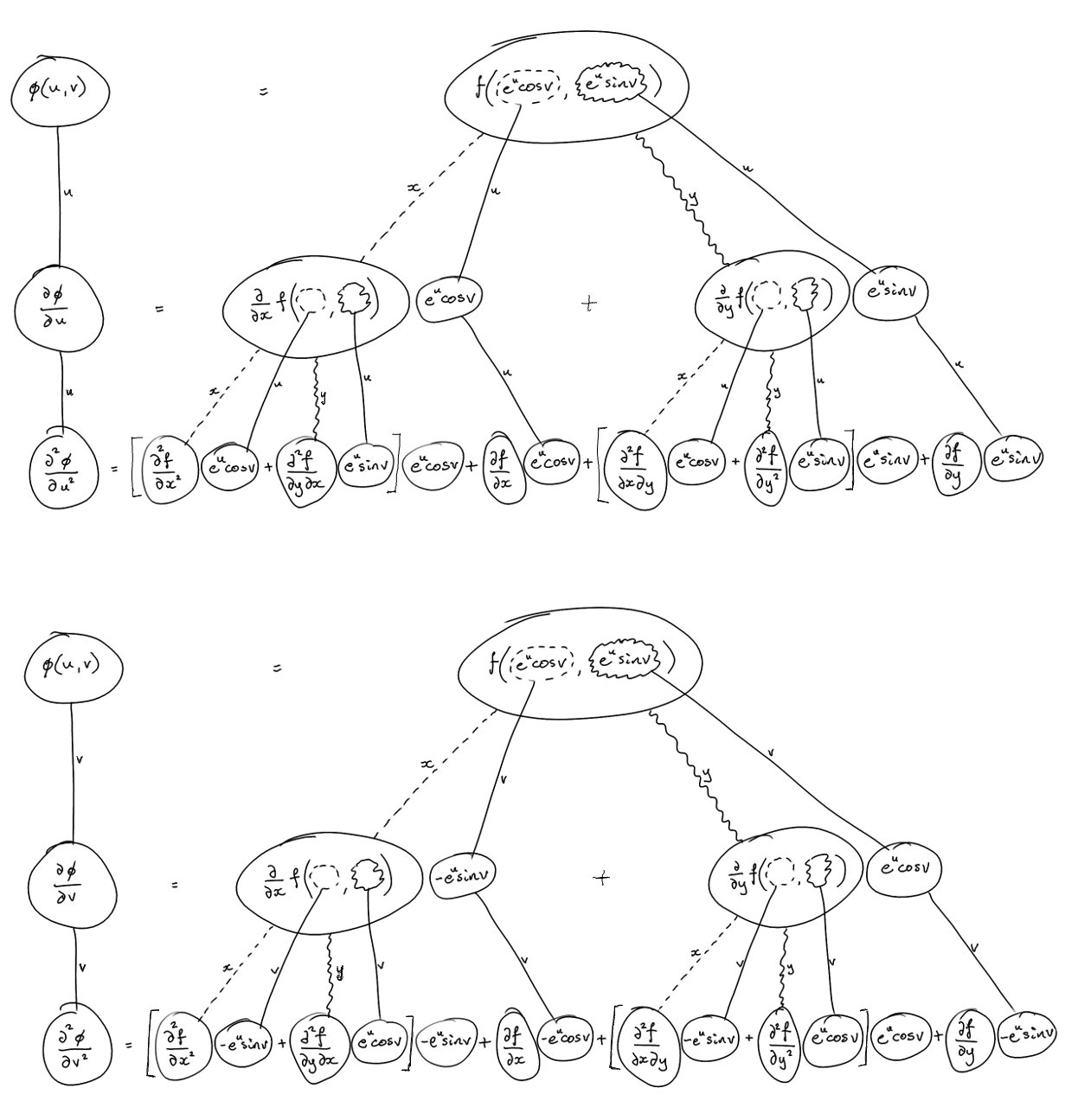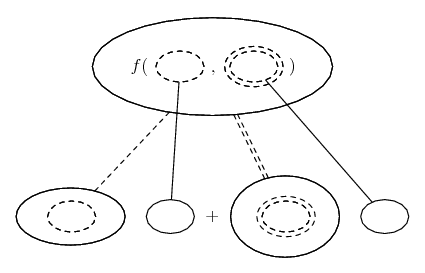# Thread: A Partial Derivatives Proof Question.

1. ##A Partial Derivatives Proof Question.

I don't know what the train of thought is to working through this proof is, could somebody please shed some light on how I should go about proving this.

All those partial derivatives look daunting and strange, I can assure you my algebra and high school calculus skills are decent, but this question just doesn't make any sense to me.

Thank you for helping me out I really do appreciate it.

2. ## Re: A Partial Derivatives Proof Question.

In case a picture helps...... where (key in spoiler) ...

Spoiler:is the chain rule for two inner functions, i.e...

$\displaystyle \displaystyle{\frac{d}{du}\ f(x(u), y(u)) = \frac{\partial f}{\partial x} \frac{dx}{du} + \frac{\partial f}{\partial y} \frac{dy}{du}}$

As with...... the ordinary chain rule, straight continuous lines differentiate downwards (integrate up) with respect to the main variable (in this case u and v in turn), and the straight dashed line similarly but with respect to the (corresponding) dashed balloon expression which is (one of) the inner function(s) of the composite expression (in this case x and y).

But for the second derivative the chain rule is wrapped inside the left leg of each of two occurances of the legs-uncrossed version of...... the product rule, where, again, straight continuous lines are differentiating downwards with respect to u or v.

Add the bottom rows and simplify.

_________________________________________

Don't integrate - balloontegrate!

Balloon Calculus; standard integrals, derivatives and methods

Balloon Calculus Drawing with LaTeX and Asymptote!

#### Search Tags

derivatives, partial, proof, question